## FractionsFraction
Fraction refers to a part of a whole.

You will encounter fractions when asked to make calculations with the TI-30XS MultiView Scientific Calculator using the on-screen mathematics formula sheet.

When asked to convert an answer from a fraction to decimal form,
use the toggle button.

Proper Fraction
Proper Fraction refers to a fraction in which the top number (numerator) is smaller than the bottom number (denominator).

Example
One Half$\bf\displaystyle\frac{1}{2}$is the proper fraction command.

A calculation involving a proper fraction produces an answer in fraction form.

Improper Fraction
Improper Fraction refers to a fraction in which the top number (numerator) is larger than the bottom number (denominator).

Example
Three Halves$\bf\displaystyle\frac{3}{2}$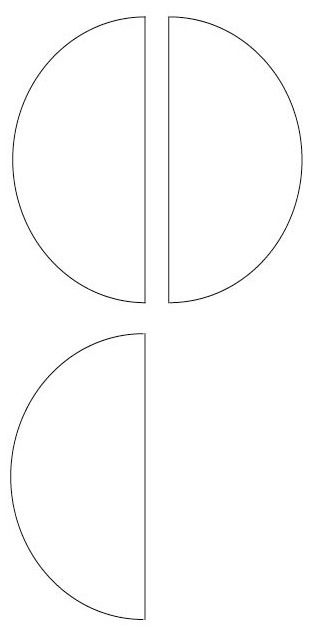is the improper fraction command.

A calculation involving an improper fraction produces an answer in fraction form.

Mixed Fraction
Mixed Fraction (also known as Mixed Number) refers to a combination of a whole number and a fraction.

Example
One and One Half$\bf\displaystyle1\frac{1}{2}$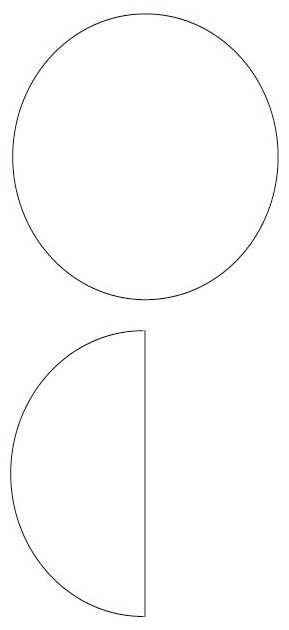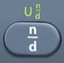is the mixed number command.activatesabove.

A calculation involving a mixed fraction produces an answer in fraction form.

Question

A small rectangular piece of cloth measures$\bf\displaystyle\frac{1}{2}$ inch long by$\bf\displaystyle\frac{1}{4}$ inch wide.  What is its area?$\bf\displaystyle\frac{1}{8}$ square inch

See Rectangle.
Area = length × width
A = lw
A =$\bf\displaystyle\frac{1}{2}$ ×$\bf\displaystyle\frac{1}{4}$$\bf\displaystyle\frac{1}{8}$

Calculator Click What You See Commentblinker clears screen1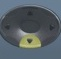2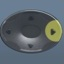×14$\bf\displaystyle\frac{1}{2}$$\bf\displaystyle\frac{1}{4}$ A = lw$\bf\displaystyle\frac{1}{8}$ Answer
###### Proper Fraction

Question
A slightly larger rectangular piece of cloth measures$\bf\displaystyle\frac{11}{4}$ inch long by$\bf\displaystyle\frac{3}{4}$ inch wide.  What is its area?$\bf\displaystyle\frac{33}{16}$ square inches

See Rectangle.
Area = length × width
A = lw
A =$\bf\displaystyle\frac{11}{4}$ ×$\bf\displaystyle\frac{3}{4}$$\bf\displaystyle\frac{33}{16}$

Calculator Click What You See Commentblinker clears screen114×34$\bf\displaystyle\frac{11}{4}$$\bf\displaystyle\frac{3}{4}$ A = lw$\bf\displaystyle\frac{33}{16}$ Answer
###### Improper Fraction

Question
A much larger piece of cloth measures 7 ½ inch by 8 ¼ inch.  What is its area?$\bf\displaystyle\frac{495}{8}$ square inches

See Rectangle.
Area = length × width
A = lw
A =$\bf\displaystyle7\frac{1}{2}$ ×$\bf\displaystyle8\frac{1}{2}$ =$\bf\displaystyle\frac{495}{8}$

Calculator Click What You See Commentblinker clears screen712×814$\bf\displaystyle7\frac{1}{2}$$\bf\displaystyle8\frac{1}{2}$ A = lw$\bf\displaystyle\frac{495}{8}$ Answer
###### Mixed Fraction

Question

With reference to the question above, express your answer in decimal form.

61.875 square inches

See Toggle.$\bf\displaystyle\frac{495}{8}$ ⇔ 61.875

Calculator Click What You See Commentblinker clears screen712×814$\bf\displaystyle7\frac{1}{2}$$\bf\displaystyle8\frac{1}{2}$ A = l × w$\bf\displaystyle\frac{495}{8}$61.875 Answer
(Toggle)
###### Fraction ⇔ Decimal

Question

With reference to the question above, round your answer to the nearest tenth.

61.9 square inches

See Rounding.
61.875  61.9

Practice – Questions
1.  A computer component is shaped like a parallelogram (Area = base × height).
It measures$\bf\displaystyle\frac{3}{4}$ centimeter at its base and$\bf\displaystyle\frac{1}{4}$ cm in height.  What is its area?

2.  A computer component is shaped like a parallelogram (Area = base × height).
It measures$\bf\displaystyle\frac{12}{7}$ centimeter at its base and$\bf\displaystyle\frac{1}{4}$ cm in height.  What is its area?

3.  A computer component is shaped like a parallelogram (Area = base × height).
It measures$\bf\displaystyle2\frac{1}{2}$ centimeter at its base and$\bf\displaystyle\frac{3}{4}$ cm in height.  What is its area?

4.  A computer component is shaped like a parallelogram (Area = base × height).
It measures$\bf\displaystyle7\frac{1}{2}$ centimeter at its base and$\bf\displaystyle5\frac{3}{4}$ cm in height.  What is its area?

5.  With respect to question 4, what is the area in decimal form rounded to the nearest hundredth?

1.$\bf\displaystyle\frac{3}{16}$ square centimeters
2.$\bf\displaystyle\frac{3}{7}$ square centimeters
3.$\bf\displaystyle\frac{15}{8}$ square centimeters
4.$\bf\displaystyle\frac{345}{8}$ square centimeters